# GRE Math : How to find the amount of rational numbers between two numbers

## Example Questions

### Example Question #1 : How To Find The Amount Of Rational Numbers Between Two Numbers

In terms of, what is the arithmetic mean of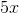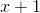, and?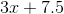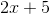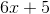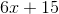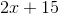Explanation:

The arithmetic mean of a set is defined as the sum of terms in the set divided by the number of terms of a set. Therefore, the mean for this problem can be found as: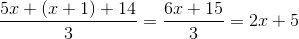### Example Question #2 : How To Find The Amount Of Rational Numbers Between Two Numbers

Harold is drawing numbered slips of paper from a hat that are labeledthrough.  What is the probability that he'll draw three numbers in a row that are divisible by three, given that a slip is not replaced after it is drawn?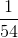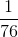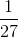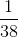Explanation:

There areslips of paper that have a number divisible by, which are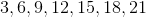The probability of one being drawn is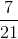. Once that slip is drawn, the probability of drawing a number divisible bybecomes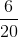, and on the third draw it is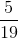.

For this sequence of events to happen, the probability is the product of the three separate probabilities: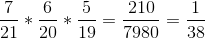### Example Question #3 : How To Find The Amount Of Rational Numbers Between Two Numbers

Happy Harold's Creamery offers specials during the week on particular sundaes. Monday they offer the Triple Trouble, in which three different scoops may be chosen from the full thirty-one that HHC offers. Tuesday features, for the same price, the Fearsome Four, which is a sundae of four scoops; however, only twenty-one flavors may be chosen from.

Quantity A: The amount of sundae options available for the Triple Trouble.

Quantity B: The amount of sundae options available for the Fearsome Four.

The relationship between the two cannot be determined.

Quantity B is greater.

The two quantities are equal.

Quantity A is greater.

Quantity B is greater.

Explanation:

Both of these sundae options are dealing with combinations, i.e. the order the scoops are chosen does not matter, and so the amount of possible combos is given by the formula: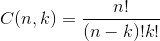Whereis the total number of options andis the size of the combination created.

For the Triple Trouble, the total amount of possible sundaes is: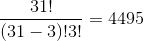And for the Fearsome Four: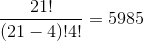### Example Question #1 : How To Find The Amount Of Rational Numbers Between Two Numbers

A shelf hasshirts, each a different pattern.

Quantity A: The total number of possible combinations of shirts ifshirts are drawn.

Quantity B: The total number of possible combinations of shirts ifshirts are drawn.

The relationship cannot be determined.

Quantity A is greater.

The two quantities are equal.

Quantity B is greater.

The two quantities are equal.

Explanation:

It is important to realize that this problem deals with combinations, rather than permutations; the order the shirts are drawn does not matter. That being said, the formula for a combination is given as:Whereis the total number of options andis the size of the combination created.

Quantity A: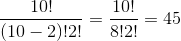Quantity B: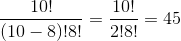### Example Question #5 : How To Find The Amount Of Rational Numbers Between Two Numbers

On a trip to his friend Leslie's house, Ron drivesmiles per hour forhours andmiles per hour forhours. What was his average speed in miles per hour for the trip?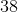Explanation:

To solve this problem, you may find the total distance traveled and divide that by the total time taken.  The distance traveled in miles is: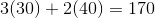Since the trip took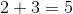hours in total, Ron's average speed in miles per hour is: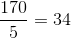### Example Question #6 : How To Find The Amount Of Rational Numbers Between Two Numbers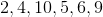Quantity A: The average of the numbers above.

Quantity B: The median of the numbers above

The two quantities are equal.

Quantity B is greater.

The relationship between the two cannot be determined.

Quantity A is greater.

Quantity A is greater.

Explanation:

The average, or arithmetic mean, of a set can be found by dividing the sum of the set by the amount of values in the set: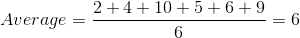The median for a set depends on if there's an even or odd number of values in the set.

For an odd number of values in a set that is arranged in numerical order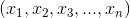, the median is given as the middle value,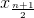.

For an even number of values in a set that is again arranged in numerical order,,there is no value exactly in the middle; rather there are two. The median is then given as the average of these two values: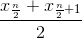Rearranging the set into numerical order gives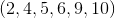, thus the median is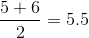Tired of practice problems?

Try live online GRE Math prep today.